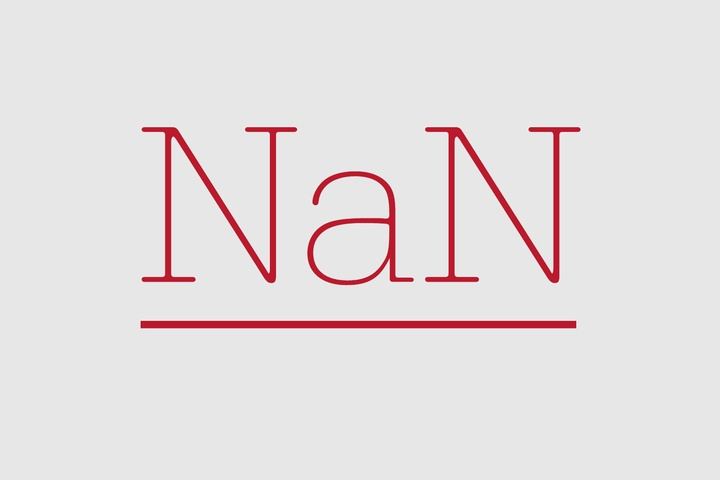Python 数据科学手册(抢先版)

#### Pandas中缺失数据的处理

Pandas对于如何处理缺失值的选择受限于它对于NumPy包的依赖。NumPy中没有对于非浮点数数据类型缺失值的内置表示。

Pandas本可以像R语言一样为每一个单独的数据类型使用特定的位模式来表示空值，但是这一方法被证明在Pandas中是相当笨重且难以实现。R语言仅包含四中基本数据类型，但NumPy支持的远多于这些：比如说，R语言只有一个整数类型，但是如果考虑到可用精度、符号和编码，NumPy支持十四种基本整数类型。如果为所有的NumPy中的数据类型都保留一个特定的位模式，这将会导致对各种数据类型的各种特定场景的操作的开销大大增加，并且其实现很有可能需要新建一个NumPy包。

NumPy是支持掩码数组的，比如说数组中附有一个单独的布尔掩码数组用来将数据标记为“好”和“坏”。Pandas本来可以沿用这一特性，但是存储、计算和代码维护上的开销使得这一选择根本没有吸引力。

#### None：Python式的缺失数据处理

Pandas首先使用的哨兵值是None。None是一个Python中的单例对象，在Python的代码中经常被用于表示缺失的数据。由于这是一个Python对象，它不是能被NumPy/Pandas中随意一个数组使用，它只能在数据类型为“Object”的数据中使用。（比如说类型为Python对象的数组）

```import numpy as np
import pandas as pd
```
```vals1 = np.array([1, None, 3, 4])
vals1
```

```for dtype in ['object', 'int']:
print("dtype =", dtype)
%timeit np.arange(1E6, dtype=dtype).sum()
print()
```

```vals1.sum()
```

#### NaN: 缺失的数值型数据

```vals2 = np.array([1, np.nan, 3, 4])
vals2.dtype
```

`1 + np.nan`
`0 *  np.nan`

`vals2.sum(), vals2.min(), vals2.max()`

```data = pd.Series([1, np.nan, 2, None])
data
```

```x = pd.Series(range(2), dtype=int)
x = None
x
```

 类型 当存储缺失数据时的类型转换 缺失数据对应的哨兵值 floating浮点值 无改变 np.nan Objcet 对象 无改变 None 或者 np.nan Integer整型 转化为 64位浮点数float64 np.nan Boolean布尔值 转化为 对象Objcet None 或者 np.nan

• isnull(): 生成一个布尔掩码数组来标示出缺失的值

• notnull(): 与isnull（）的作用相反

• dropna(): 返回一个过滤缺失值/空值后的数据

• fillna(): 返回一个数据拷贝，其中的缺失值都已被填充或者估算

#### 检测空值

Pandas数据结构中有两种检测空数据的有用的方法：isnull() 以及 notnull()。

```data = pd.Series([1, np.nan, 'hello', None])
```
```data.isnull()
```

```data[data.notnull()]
```

isnull() 和 notnull() 对于DataFrame会产生相似的布尔结果。

`data.dropna()`

```df = pd.DataFrame([[1, np.nan, 2],
[2, 3, 5],
[np.nan, 4, 6]])
df
```

`df.dropna()`

`df.dropna(axis=1)`

how参数的默认值是‘any’，使得任何包含空值的行或者列（取决于轴关键字）将会被剔除。你也可以将how指定为‘all’，这样的话只有所有值都为空的行和列才会被去除。

```df = np.nan
df
```
```df.dropna(axis=1, how='all')
```

```df.dropna(thresh=3)
```

#### 填充空值

Consider the following Series:参考下面这个Series：

```data = pd.Series([1, np.nan, 2, None, 3], index=list('abcde'))</p>
data
```

`data.fillna(0)`

```# forward-fill
data.fillna(method='fill')
```

```# back-fill
data.fillna(method='bill')
```

```df
```
`df.fillna(method='ffill', axis=1)`Not a number